###### eISSN: 2576-4500# Aeronautics and Aerospace Open Access JournalResearch Article Volume 2 Issue 5

# Finite element analysis of flow and heat transfer of dissipative Casson-Carreau nanofluid over a stretching sheet embedded in a porous medium

#### MG Sobamowo, function clickButton(){ var name=document.getElementById('name').value; var descr=document.getElementById('descr').value; var unCopyslNo=document.getElementById('unCopyslNo').value; document.getElementById("mydiv").style.display = "none"; $.ajax({ type:"post", url:"https://medcraveonline.com/captchaCode/server_action", data: { 'name' :name, 'descr' :descr, 'unCopyslNo': unCopyslNo }, cache:false, success: function (html) { //alert('Data Send');$('#msg').html(html); } }); return false; } Verify Captcha Regret for the inconvenience: we are taking measures to prevent fraudulent form submissions by extractors and page crawlers. Please type the correct Captcha word to see email ID.function refreshPage(){ $("#mydiv").load(location.href + " #mydiv"); }$(document).ready(function () { //Disable cut copy paste $('#msg').bind('cut copy paste', function (e) { e.preventDefault(); }); //Disable mouse right click$("#msg").on("contextmenu",function(e){ return false; }); }); .noselect { -webkit-touch-callout: none; /* iOS Safari */ -webkit-user-select: none; /* Safari */ -khtml-user-select: none; /* Konqueror HTML */ -moz-user-select: none; /* Firefox */ -ms-user-select: none; /* Internet Explorer/Edge */ user-select: none; /* Non-prefixed version, currently supported by Chrome and Opera */ cursor: none; } AA Yinusa, AA Oluwo, SI Alozie

Department of Mechanical Engineering, University of Lagos, Nigeria

Correspondence: MG Sobamowo, Department of Mechanical Engineering, University of Lagos, Akoka, Lagos, Nigeria, Tel 2347034717417

Received: September 18, 2018 | Published: October 8, 2018

Citation: Sobamowo MG, Yinusa AA, OluwoAA, et al. Finite element analysis of flow and heat transfer of dissipative Casson-Carreau nanofluid over a stretching sheet embedded in a porous medium. Aeron Aero Open Access J. 2018;2(5):294-308. DOI: 10.15406/aaoaj.2018.02.00064

# Abstract

In this paper, combined influences of thermal radiation, inclined magnetic field and temperature-dependent internal heat generation on unsteady two-dimensional flow and heat transfer analysis of dissipative Casson-Carreau nanofluid over a stretching sheet embedded in a porous medium is investigated. Similarity transformations are used to reduce the developed systems of governing partial differential equations to nonlinear third and second orders ordinary differential equations which are solved using finite element method. In the study, kerosene is used as the base fluid which is embedded with the silver (Ag) and copper (Cu) nanoparticles. Also, effects of other pertinent parameters on the flow and heat transfer characteristics of the Casson-Carreau nanofluids are investigated and discussed. From the results, it is established temperature field and the thermal boundary layers of Ag-Kerosene nanofluid are highly effective when compared with the Cu-Kerosene nanofluid. Heat transfer rate is enhanced by increasing in power-law index and unsteadiness parameter. Skin friction coefficient and local Nusselt number can be reduced by magnetic field parameter and they can be enhanced by increasing the aligned angle. Friction factor is depreciated and the rate of heat transfer increases by increasing the Weissenberg number. A very good agreement is established between the results of the present study and the previous results. The results of present analysis can help in expanding the understanding of the thermo-fluidic behaviour of the Casson-Carreau nanofluid over a stretching sheet as applied in manufacturing industries and production engineering.

Keywords: MHD, nanofluid, non-uniform heat source/sink, Carreau fluid, thermal radiation and free convection, finite element method

# Introduction

The recent studies in the past few decades have shown that the study of Non-Newtonian have attracted tremendous attractions in the study of fluid dynamics. The flow applications of non-Newtonian fluids are evident in polymer devolatization and processing, wire and fiber coating, heat exchangers, extrusion process, chemical processing equipment, etc. Also, combining heat transfer with the concept of stretching flow is vital in the afore-mentioned areas of applications. Such processes have great affinity for cooling rates and stretching simultaneously.1 Consequently, in the past few years, research efforts have been directed towards the analysis of this very important phenomenon of wide areas of applications. Moreover, the promising significance of magnetohydrodynamics (MHD) fluid behavior in concerned applications such as in blood ﬂow still provokes the continuous studies and interests of researchers. Additionally, the incorporation of radiation through thermal analysis is vital in technology involving solar energy, space vehicles, systems with propulsion, plasma physics in the flow structure of atomic plants, combustion processes, internal combustion engines, ship compressors, solar radiations and in chemical processes and space ship with high temperature level re-entry aerodynamics. Furthermore, there are various engineering and industrial applications of magnetohydrodynamics (MHD) fluid behavior such as in the study of the growth of crystals, blood ﬂow etc. Therefore, the influences of external factors such as thermal radiation and magnetic field on the thermofluid problem concerning Newtonian and Non-Newtonian fluid have been widely analyzed in recent times.

In an early study, MHD fluid flow over a surface that is susceptible to stretching was critically studied by Anderson et al.2,3 In their studies, effect of transient variables on the film size2 and magnetic influence on the fluid flow characteristics were explored numerically.3 Few years later, Chen4 investigated the fluid film that obeys power-law flow of unsteady thermal-stretching sheet while Dandapat5,6 focused on the effect of changing viscosity as well as thermo-capillarity on the heat transfer rate of film flow over a sheet susceptible to stretching. Meanwhile, Wang7 developed an analytical or close form solution for momentum and energy (heat transfer) of film flow over a surface susceptible to stretching. Also, Chen8 and Sajid et al.9 investigated the motion characteristics involving non-Newtonian thin film over a transient stretching surface considering viscous dissipation using HAM and HPM. After a year, Dandapat et al.,10 presented the analysis using a two-dimensional flow over a transient sheet that is capable of stretching while in the same year, impact of power-law index was carried out by Abbasbandy et al.11 Santra & Dandapat12 numerically investigated the same considering a transient horizontal elongating sheet. A numerical approach was also used by Sajid et al.13 to analyze the micropolar film flow over an inclined plate, moving belt and vertical cylinder. A year later, Noor & Hashim14 investigated the influence of magnetic parameter and thermocapillarity on an unsteady liquid film flow over similar sheet while Dandapat & Chakraborty15 and Dandapat & Singh16 presented the thin film flow analysis over a non-linear stretching surface with the effect of transverse magnetic field. Heat transfer characteristics of the thin film flows considering the different channels have also been analyzed by Abdel-Rahman,17 Khan et al.18 Liu et al.19 and Vajaravelu et al.20 Meanwhile, Liu & Megahad21 used HPM to analyze the thermofluidic effect of thin film with internal heat generation and changing heat flux while Seddeek as well as other recent works22-35 investigated the impacts of thermal radiation and changing viscosity on magnetohydrodynamics in unforced convection fluid ﬂow over a ﬂat plate that is semi-inﬁnite.

Other researchers went on to present their view on the interesting topic by adapting nanofluid into the generic three dimensional fluid model with radiation effect.36-48 Makinde & Animasaun49 investigated the effect of cross diffusion on MHD bioconvection flow over a horizontal surface. In another study, Makinde & Animasaun50 presented the MHD nanofluid on bioconvection flow of a paraboloid revolution with nonlinear thermal radiation and chemical reaction while Sandeep,51 Reddy et al.52 and Ali et al.53 studied the heat transfer behaviour of MHD flows. Maity et al.54 analyzed thermocapillary flow of a thin Nanoliquid film over an unsteady stretching sheet. Furthermore, different studies on the flow and heat transfer behaviour as well as entropy generation for different non-Newtonian fluids under difference internal and external conditions.55-72 The above reviewed studies have been the consequent of the various industrial and engineering applications of non-Newtonian fluids. Among the classes of non-Newtonian fluids, Carreau fluid which its rheological expressions were first introduced by Carreau,73 is one of the non-Newtonian fluids that its model is substantial for gooey, high and low shear rates.74 On account of this headway, it has profited in numerous innovative and assembling streams.74-87 Owing to these applications, different studies have been carried out to explore the characteristics of Carreau liquid in ﬂow under different conditions. Kumar et al.40 applied numerical scheme to analyze the thermofluidic behaviour of a liquid film capable of conducting electricity. The fluid is based on the structure of liquid phase and interactive behaviour of solid of a two-phase suspension. It is able to capture complex rheological properties of a fluid, unlike other simplified models like the power law88 and second, third or fourth-grade models.89,90

Casson fluids are Jelly, honey, protein, Human blood and fruit juices. Concentrated fluids like sauces, honey, juices, blood, and printing inks can be well described using this model. The effect of magnetohydrodynamic Casson fluid flow on a laterally positioned elongating sheet was explained by Nadeem et al.91 The review studies have been analyzed using approximate analytical, semi-analytical and numerical methods. Among the numerical methods, the numerical solutions of FEM represent efficient ways of obtaining solutions to nonlinear problems even with complexities in the boundary conditions and geometries.92-94 Therefore, using FEM, this work presents numerical investigations of the combined influences of thermal radiation, inclined magnetic field and internal heat generation that is temperature-dependent on unsteady two-dimensional flow and heat transfer analysis of dissipative Casson-Carreau nanofluid over a stretching sheet embedded in a porous medium. Using kerosene as the base fluid embedded with the silver (Ag) and copper (Cu) nanoparticles, the effects of other pertinent parameters on flow and heat transfer characteristics of the nanofluids are investigated and discussed. The analysis of the stretched flows with heat transfer is very significant in controlling the qualities of the end products in manufacturing and metal forming processes. Such processes have great dependences on the stretching and cooling rates. The results of present analysis can help in expanding the understanding of the thermo-fluidic behaviour of the Casson-Carreau nanofluid over a stretching sheet as applied in manufacturing industries and production engineering.

# Problem formulation

From the transient, two-dimensional boundary layer fluid flow of a conducting and heat generating Casson and Carreau nanofluids over a sheet susceptible to stretching extreme by liquid film of uniform size (thickness) h(t) as represented schematically in Figure 1. The stretching velocity along x-axis is U(x,t) and y–axis is perpendicular to it with dissipation and volume fraction considered.

Using ,

$\tau ={\tau }_{0}+\mu \stackrel{˙}{\sigma }$           (1)

or

(2)

Also employing the tensor given as95

${\overline{\tau }}_{ij}={\eta }_{0}\left[1+\frac{\left(n-1\right)}{2}{\left(\Gamma \overline{\stackrel{˙}{\gamma }}\right)}^{2}{\overline{\gamma }}_{ij}\right]$           (3)

${\overline{\tau }}_{ij}$ is the extra tensor, ηo is the zero shear rate viscosity, Γ is the time constant, n is the power-law index and $\overline{\stackrel{˙}{\gamma }}$  is defined as

$\overline{\stackrel{˙}{\gamma }}=\sqrt{\frac{1}{2}{\sum }_{i}{\sum }_{j}{\overline{\stackrel{˙}{\gamma }}}_{ij}{\overline{\stackrel{˙}{\gamma }}}_{ji}}=\sqrt{\frac{1}{2}}\Pi$

where $\Pi$  is the second invariant strain tensor.

Following the assumptions, the equations for continuity and motion for the flow analysis of Carreau and Casson fluids are

$\frac{\partial u}{\partial x}+\frac{\partial v}{\partial y}=0$          (4)

${\rho }_{nf}\left(\frac{\partial u}{\partial t}+u\frac{\partial u}{\partial x}+v\frac{\partial u}{\partial y}\right)={\mu }_{nf}\left(1+\frac{1}{\beta }\right)\left(1+\frac{3\left(n-1\right){\Gamma }^{2}}{2}{\left(\frac{\partial u}{\partial y}\right)}^{2}\right)\frac{{\partial }^{2}u}{\partial {y}^{2}}-\sigma {B}_{o}^{2}uco{s}^{2}\gamma -\frac{{\mu }_{nf}u}{K}$       (5)

${\left(\rho {C}_{p}\right)}_{nf}\left(\frac{\partial u}{\partial t}+u\frac{\partial T}{\partial x}+v\frac{\partial T}{\partial y}\right)={k}_{nf}\frac{{\partial }^{2}T}{\partial {y}^{2}}+{\mu }_{nf}{\left(\frac{\partial u}{\partial y}\right)}^{2}+{q}^{‴}-\frac{\partial {q}_{r}}{\partial y}$                 (6)

where

${\rho }_{nf}={\rho }_{f}\left(1-\varphi \right)+{\rho }_{s}\varphi$       (7a)

${\left(\rho {C}_{p}\right)}_{nf}={\left(\rho {C}_{p}\right)}_{f}\left(1-\varphi \right)+\left(\rho {C}_{p}\right)\varphi$               (7b)

${\sigma }_{nf}={\sigma }_{f}\left[1+\frac{3\left\{\frac{{\sigma }_{s}}{{\sigma }_{f}}-1\right\}\varphi }{\left\{\frac{{\sigma }_{s}}{{\sigma }_{f}}+2\right\}\varphi -\left\{\frac{{\sigma }_{s}}{{\sigma }_{f}}-1\right\}\varphi }\right]$       (7c)

${\mu }_{nf}=\frac{{\mu }_{f}}{{\left(1-\varphi \right)}^{2.5}}$    (8)

${k}_{nf}={k}_{f}\left[\frac{{k}_{s}+2{k}_{f}-2\varphi \left({k}_{f}-{k}_{s}\right)}{{k}_{s}+2{k}_{f}+\varphi \left({k}_{f}-{k}_{s}\right)}\right]$   (9)

$\frac{\partial {q}_{r}}{\partial y}=-\frac{4{\sigma }^{\ast }}{3{k}^{\ast }}\frac{\partial {T}^{4}}{\partial y}\cong -\frac{16{\sigma }^{\ast }{T}_{s}^{3}}{3{k}^{\ast }}\frac{{\partial }^{2}T}{\partial {y}^{2}}$ (using Roseland’s approximation) (10)
Assuming no slip condition, the appropriate boundary conditions are given as

$\text{\hspace{0.17em}}u={U}_{w},\text{ }v=0,\text{\hspace{0.17em}}\text{ }T={T}_{s}\text{ }at\text{ }y=0\text{ }$          (11a)

$\frac{\partial u}{\partial y}=0,\text{ }\frac{\partial T}{\partial y}=0,\text{ }y=h$    (11b)

It should be stated at this juncture that the formulated mathematical model is implicitly in the domain x≥0. In other to annul discontinuities as a result of surface effects, a further assumption of smooth surface is made. Likewise, the effect of shear due to the interfacial quiescent atmosphere i.e. is removed. The shear stress based on the Newton’s law of viscosity $\tau =\mu \frac{\partial u}{\partial y}$  as well as the heat flux $\stackrel{˙}{{q}^{″}}=-k\left(\frac{\partial T}{\partial y}\right)$ disappear when the adiabatic free surface condition is considered (at y=h).

It should be noted that

$v=\frac{dh}{dt}=-\frac{\alpha \beta }{2}{\left(\frac{{v}_{f}}{b\left(1-\alpha t\right)}\right)}^{\frac{1}{2}},\text{ }y=h\left(t\right)=-\int \left\{\frac{\alpha \beta }{2}{\left(\frac{{v}_{f}}{b\left(1-\alpha t\right)}\right)}^{\frac{1}{2}}\right\}dt$         (12)

The above boundary conditions are in line with the works of Kumar et al.,56 and Abel et al.,76

The non-uniform heat generation/absorption ${q}^{‴}$  is taken as

${q}^{‴}=\frac{{k}_{f}{U}_{w}}{x{\nu }_{f}}\left[{A}^{\ast }\left({T}_{s}-{T}_{o}\right)f\text{'}+{B}^{\ast }\left({T}_{s}-{T}_{o}\right)\right]$      (13)

where To is the ambient temperature and the surface temperature Ts of the stretching sheet varies with respect to distance x-from the slit as

${T}_{s}={T}_{o}-{T}_{ref}\left(\frac{b{x}^{2}}{2{v}_{f}{\left(1-at\right)}^{\frac{3}{2}}}\right)$             (14)

And the stretching velocity varies with respect to x as

$U=\frac{bx}{\left(1-at\right)}$         (15)

On introducing the following stream functions

$u=\frac{\partial \psi }{\partial y},\text{ }v=\frac{\partial \psi }{\partial x}$               (16)

And the similarity variables

$\begin{array}{l}u=\frac{bx}{\left(1-at\right)}{f}^{\prime }\left(\eta ,t\right),\text{ }v=-{\left(b{\nu }_{f}\right)}^{-\frac{1}{2}}{\left(1-at\right)}^{-\frac{1}{2}}f\left(\eta ,t\right),\\ \eta ={\left(b/{\nu }_{f}\right)}^{\frac{1}{2}}{\left(1-at\right)}^{-\frac{1}{2}}y,\text{ }T={T}_{o}-{T}_{ref}\left(b{x}^{2}/2{v}_{f}\right){\left(1-at\right)}^{-\frac{3}{2}}\theta \left(\eta \right)\end{array}$          (17)

Substituting Eq. (16) and (17) into Eq. (5), (6) and (7), we have a partially coupled third and second orders ordinary differential equation

$\begin{array}{l}\left(1+\frac{1}{\beta }\right){f}^{‴}\left\{1+\frac{3\left(n-1\right)We{\left({f}^{″}\right)}^{2}}{2}\right\}+{B}_{1}\left\{{B}_{2}\left(S\left({f}^{\prime }+\frac{\eta }{2}{f}^{″}\right)+f{f}^{″}-{\left({f}^{\prime }\right)}^{2}\right)\right\}\\ -H{a}^{2}{f}^{\prime }co{s}^{2}\gamma -\frac{1}{Da}{f}^{\prime }=0\end{array}$         (18a)

${B}_{3}\left(1+\frac{4}{3}R\right){\theta }^{″}+\frac{EcPr}{{B}_{1}}{\left({f}^{″}\right)}^{2}+\left({A}^{\ast }{f}^{\prime }+{B}^{\ast }\theta \right)-{B}_{4}Pr\left\{\frac{S}{2}\left(\left(\eta {\theta }^{\prime }+3\theta \right)+2{f}^{\prime }\theta -f{\theta }^{\prime }\right)\right\}=0$ (18b)

where

$\begin{array}{l}W{e}^{2}=\frac{{b}^{3}{x}^{2}{\Gamma }^{2}}{{v}_{f}{\left(1-at\right)}^{3}},\text{ }Pr=\frac{\mu {c}_{p}}{{k}_{f}},\text{ }H{a}^{2}=\frac{{\sigma }_{nf}{B}_{o}^{2}}{{\rho }_{f}b},\text{ }Ec=\frac{{U}_{w}^{2}}{{c}_{p}\left({T}_{s}-{T}_{0}\right)},\text{ }S=\frac{\alpha }{b},\text{ }R=\frac{4{\sigma }^{\ast }{T}_{0}^{3}}{{k}^{\ast }{k}_{f}}\\ {B}_{1}={\left(1-\varphi \right)}^{2.5},\text{ }{B}_{2}=1-\varphi +\varphi \frac{{\rho }_{s}}{{\rho }_{f}},\text{ }{B}_{3}=\frac{{k}_{nf}}{{k}_{f}},\text{ }{B}_{4}=1-\varphi +\varphi \frac{{\left(\rho {c}_{p}\right)}_{s}}{{\left(\rho {c}_{p}\right)}_{f}},\text{ }Da=\frac{K}{{h}_{o}}\end{array}$ (19)

And the boundary conditions become

$\text{\hspace{0.17em}}\eta =0,\text{ }f=0,\text{ }{f}^{\prime }=1,\text{ }\theta =0\text{ }$

$\eta =\beta ,\text{ }f=\frac{S\beta }{2},\text{ }{f}^{″}=0,\text{ }{\theta }^{\prime }=0\text{ }\text{ }\text{\hspace{0.17em}}$          (20)

# Method of solution: finite element method

Equations (18a) and (18b) are systems of coupled non-linear ordinary differential equations which are to be solved along side with boundary conditions in Eq. (20). The exact analytical solutions for these non-linear equations are not possible. Therefore, in order to solve the equations, recourse is made to a numerical method. In this work, finite element method is applied to analyze the systems of the coupled nonlinear equations. The variational and the finite element formulation of Eqs. (18a) and (18b) are given as follows:

In order to reduce the system of the nonlinear equation, we let

$g={f}^{\prime }\text{ }\text{\hspace{0.17em}}$ (21)

The system of equation (18a) and (18b) thus reduces to

$\begin{array}{l}\left(1+\frac{1}{\beta }\right)\left\{1+\frac{3\left(n-1\right)We{\left({g}^{\prime }\right)}^{2}}{2}\right\}{g}^{″}+{B}_{1}\left\{{B}_{2}\left(S\left(g+\frac{\eta }{2}{g}^{\prime }\right)+f{g}^{\prime }-{\left(g\right)}^{2}\right)\right\}\\ -H{a}^{2}gco{s}^{2}\gamma -\frac{1}{Da}{f}^{\prime }=0\end{array}$               (22a)

${B}_{3}\left(1+\frac{4}{3}R\right){\theta }^{″}+\frac{EcPr}{{B}_{1}}{\left({g}^{\prime }\right)}^{2}+\left({A}^{\ast }g+{B}^{\ast }\theta \right)-{B}_{4}Pr\left\{\frac{S}{2}\left(\left(\eta {\theta }^{\prime }+3\theta \right)+2g\theta -f{\theta }^{\prime }\right)\right\}=0$ (22b)

And the corresponding boundary conditions become

$\text{\hspace{0.17em}}\eta =0,\text{ }f=0,\text{ }g=1,\text{ }\theta =0\text{ }$

$\eta =\beta ,\text{ }f=\frac{S\beta }{2},\text{ }{g}^{\prime }=0,\text{ }{\theta }^{\prime }=0\text{ }\text{ }\text{\hspace{0.17em}}$           (23)

The variation forms associated with the Eqs. (21), (22a) and (22b) over a typical two-nodal linear element $\left({\eta }_{e},\text{\hspace{0.17em}}\text{\hspace{0.17em}}{\eta }_{e+1}\right)$  are given as follows:

${\int }_{{\eta }_{e}}^{{\eta }_{e+1}}{w}_{1}\left[{f}^{\prime }-g\right]d\eta =0\text{ }\text{\hspace{0.17em}}$     (24)

${\int }_{{\eta }_{e}}^{{\eta }_{e+1}}{w}_{2}\left[\begin{array}{l}\left(1+\frac{1}{\beta }\right)\left\{1+\frac{3\left(n-1\right)We{\left({g}^{\prime }\right)}^{2}}{2}\right\}{g}^{″}+{B}_{1}\left\{{B}_{2}\left(S\left(g+\frac{\eta }{2}{g}^{\prime }\right)+f{g}^{\prime }-{\left(g\right)}^{2}\right)\right\}\\ -H{a}^{2}gco{s}^{2}\gamma -\frac{1}{Da}g\end{array}\right]d\eta =0$ (25)

${\int }_{{\eta }_{e}}^{{\eta }_{e+1}}{w}_{3}\left[{B}_{3}\left(1+\frac{4}{3}R\right){\theta }^{″}+\frac{EcPr}{{B}_{1}}{\left({g}^{\prime }\right)}^{2}+\left({A}^{\ast }g+{B}^{\ast }\theta \right)-{B}_{4}Pr\left(\frac{S}{2}\left(\left(\eta {\theta }^{\prime }+3\theta \right)+2g\theta -f{\theta }^{\prime }\right)\right)\right]d\eta =0$ (26)

where w1, wsub>2 and wsub>3 are the weight functions or variational in f, g and θ, respectively.

The Galerkin finite element formulation may be obtained from Eq. (24)-(25) by substituting the finite element approximations of the form:

$f=\sum _{j=1}^{2}{N}_{j}{f}_{j},\text{\hspace{0.17em}}\text{ }g=\sum _{j=1}^{2}{N}_{j}{g}_{j},\text{ }\theta =\sum _{j=1}^{2}{N}_{j}{\theta }_{j}.$     (27)

For the Galerkin finite element formulation, the weight function is equal to the basis/shape/interpolation function. Therefore, w1=w2=w3=Ni(i=1,2), where Ni are the basis/shape/interpolation functions when considering the linear element $\left({\eta }_{e},\text{\hspace{0.17em}}\text{\hspace{0.17em}}{\eta }_{e+1}\right)$ which are defined as follows:

${N}_{1}=\frac{{\eta }_{e+1}-\text{\hspace{0.17em}}\eta }{{\eta }_{e+1}-\text{\hspace{0.17em}}{\eta }_{e}},\text{ }{N}_{2}=\frac{\eta -{\eta }_{e}}{{\eta }_{e+1}-\text{\hspace{0.17em}}{\eta }_{e}},\text{ }{\eta }_{e}\le \eta \le {\eta }_{e+1}$           (28)

Therefore, the equivalent Galerkin finite element formulations of Eqs. (24)-(26) are

${\int }_{{\eta }_{e}}^{{\eta }_{e+1}}{N}_{i}\left[{f}^{\prime }-g\right]d\eta =0\text{ }\text{\hspace{0.17em}}$    (29)

${\int }_{{\eta }_{e}}^{{\eta }_{e+1}}{N}_{i}\left[\begin{array}{l}\left(1+\frac{1}{\beta }\right)\left\{1+\frac{3\left(n-1\right)We{\left({g}^{\prime }\right)}^{2}}{2}\right\}{g}^{″}+{B}_{1}\left\{{B}_{2}\left(S\left(g+\frac{\eta }{2}{g}^{\prime }\right)+f{g}^{\prime }-{\left(g\right)}^{2}\right)\right\}\\ -H{a}^{2}gco{s}^{2}\gamma -\frac{1}{Da}g\end{array}\right]d\eta =0$ (30)

${\int }_{{\eta }_{e}}^{{\eta }_{e+1}}{N}_{i}\left[{B}_{3}\left(1+\frac{4}{3}R\right){\theta }^{″}+\frac{EcPr}{{B}_{1}}{\left({g}^{\prime }\right)}^{2}+\left({A}^{\ast }g+{B}^{\ast }\theta \right)-{B}_{4}Pr\left(\frac{S}{2}\left(\left(\eta {\theta }^{\prime }+3\theta \right)+2g\theta -f{\theta }^{\prime }\right)\right)\right]d\eta =0$ (31)

Incorporating the boundary conditions directly in the strong forms as presented Eqs. (30) and (32) is a daunting task. Also, the requirement on continuity of field variables is much stronger in its present strong forms. In order to overcome the difficulties, weak formulations are preferred. Indisputably, the weak formulations help to reduce the order of continuity needed for elements selected i.e. it will reduce the continuity requirements on the approximation (or basis functions) functions thereby allowing the use of easy-to-construct and implement polynomials. Moreover, weak formulation automatically enforces natural boundary conditions.

The weak formulations of Eqs. (30) and (31) are

$\begin{array}{l}\left(1+\frac{1}{\beta }\right)\left\{{{N}_{i}{g}^{\prime }|}_{{\eta }_{e}}^{{\eta }_{e+1}}-{\int }_{{\eta }_{e}}^{{\eta }_{e+1}}{g}^{\prime }{N}^{\prime }d\eta +\frac{\left(n-1\right)We}{2}\left[{{N}_{i}{\left({g}^{\prime }\right)}^{3}|}_{{\eta }_{e}}^{{\eta }_{e+1}}-{\int }_{{\eta }_{e}}^{{\eta }_{e+1}}{\left({g}^{\prime }\right)}^{3}{N}^{\prime }d\eta \right]\right\}\\ +{\int }_{{\eta }_{e}}^{{\eta }_{e+1}}{N}_{i}\left[{B}_{1}\left\{{B}_{2}\left(S\left(g+\frac{\eta }{2}{g}^{\prime }\right)+f{g}^{\prime }-{\left(g\right)}^{2}\right)\right\}-H{a}^{2}gco{s}^{2}\gamma -\frac{1}{Da}g\right]d\eta =0\text{ }\text{\hspace{0.17em}}\end{array}$       (32)

${B}_{3}\left(1+\frac{4}{3}R\right)\left[{{N}_{i}{\theta }^{\prime }|}_{{\eta }_{e}}^{{\eta }_{e+1}}-{\int }_{{\eta }_{e}}^{{\eta }_{e+1}}{\theta }^{\prime }{N}^{\prime }d\eta \right]+{\int }_{{\eta }_{e}}^{{\eta }_{e+1}}{N}_{i}\left[\begin{array}{l}\frac{EcPr}{{B}_{1}}{\left({g}^{\prime }\right)}^{2}+\left({A}^{\ast }g+{B}^{\ast }\theta \right)\\ -{B}_{4}Pr\left(\frac{S}{2}\left(\left(\eta {\theta }^{\prime }+3\theta \right)+2g\theta -f{\theta }^{\prime }\right)\right)\end{array}\right]d\eta =0$ (33)

Note that Eq. (29) has already been expressed in a weak form.

Substituting Eq. (27) into Eqs. (24), (32) and (33), we have

${\int }_{{\eta }_{e}}^{{\eta }_{e+1}}{N}_{i}\left[\sum _{i=1}^{2}{N}_{j}^{\text{'}}{f}_{j}-\sum _{j=1}^{2}{N}_{j}{g}_{j}\right]d\eta =0\text{ }\text{\hspace{0.17em}}$              (34)

$\begin{array}{l}\left(1+\frac{1}{\beta }\right)\left\{{{N}_{i}\sum _{i=1}^{2}{N}_{j}^{\text{'}}{g}_{j}|}_{{\eta }_{e}}^{{\eta }_{e+1}}-{\int }_{{\eta }_{e}}^{{\eta }_{e+1}}\sum _{i=1}^{2}{N}_{j}^{\text{'}}{g}_{j}{N}_{i}^{\text{'}}d\eta +\left(\frac{\left(n-1\right)We}{2}\right)\left[{{N}_{i}{\left(\sum _{i=1}^{2}{N}_{j}^{\text{'}}{g}_{j}\right)}^{3}|}_{{\eta }_{e}}^{{\eta }_{e+1}}-{\int }_{{\eta }_{e}}^{{\eta }_{e+1}}{\left(\sum _{i=1}^{2}{N}_{j}^{\text{'}}{g}_{j}\right)}^{3}{N}_{i}^{\text{'}}d\eta \right]\right\}\\ +{\int }_{{\eta }_{e}}^{{\eta }_{e+1}}{N}_{i}\left[{B}_{1}\left\{{B}_{2}\left(\begin{array}{l}S\left(\sum _{i=1}^{2}{N}_{j}{g}_{j}+\frac{\eta }{2}\sum _{i=1}^{2}{N}_{j}^{\text{'}}{g}_{j}\right)\\ +\left(\sum _{i=1}^{2}{N}_{j}{f}_{j}\cdot \sum _{i=1}^{2}{N}_{j}^{\text{'}}{g}_{j}\right)-{\left(\sum _{i=1}^{2}{N}_{j}{g}_{j}\right)}^{2}\end{array}\right)\right\}-H{a}^{2}\sum _{i=1}^{2}{N}_{j}{g}_{j}co{s}^{2}\gamma -\frac{1}{Da}\sum _{i=1}^{2}{N}_{j}{g}_{j}\right]d\eta =0\text{ }\text{\hspace{0.17em}}\end{array}$              (35)

$\begin{array}{l}{B}_{3}\left(1+\frac{4}{3}R\right)\left\{\left[{{N}_{i}\sum _{j=1}^{2}{N}_{j}^{\text{'}}{\theta }_{j}^{\text{'}}|}_{{\eta }_{e}}^{{\eta }_{e+1}}-{\int }_{{\eta }_{e}}^{{\eta }_{e+1}}\sum _{i=1}^{2}{N}_{j}^{\text{'}}{\theta }_{j}{N}_{i}^{\text{'}}d\eta \right]\right\}\\ +{\int }_{{\eta }_{e}}^{{\eta }_{e+1}}{N}_{i}\left[\begin{array}{l}\frac{EcPr}{{B}_{1}}{\left(\sum _{i=1}^{2}{N}_{j}^{\text{'}}{g}_{j}\right)}^{2}+\left({A}^{\ast }\left(\sum _{i=1}^{2}{N}_{j}{g}_{j}\right)+{B}^{\ast }\sum _{j=1}^{2}{N}_{j}{\theta }_{j}\right)\\ -{B}_{4}Pr\left(\frac{S}{2}\left(\begin{array}{l}\left(\eta \sum _{j=1}^{2}{N}_{j}^{\text{'}}{\theta }_{j}+3\sum _{j=1}^{2}{N}_{j}{\theta }_{j}\right)+2\left(\sum _{i=1}^{2}{N}_{j}{g}_{j}\cdot \sum _{j=1}^{2}{N}_{j}{\theta }_{j}\right)\\ -{f}_{j}\sum _{j=1}^{2}{N}_{j}^{\text{'}}{\theta }_{j}\end{array}\right)\right)\end{array}\right]d\eta =0\end{array}$ (36)

A linearized analysis of the above equations can be performed if Eqs .(35) and (36) are linearized by incorporating the functions $\overline{f}$ and $\overline{\text{g}}$ , which are assumed to be known. Therefore, we arrived at

$\begin{array}{l}\left(1+\frac{1}{\beta }\right)\left\{{{N}_{i}\sum _{i=1}^{2}{N}_{j}^{\text{'}}{g}_{j}|}_{{\eta }_{e}}^{{\eta }_{e+1}}-{\int }_{{\eta }_{e}}^{{\eta }_{e+1}}\sum _{i=1}^{2}{N}_{j}^{\text{'}}{g}_{j}{N}_{i}^{\text{'}}d\eta +\left(\frac{\left(n-1\right)We}{2}\right)\left[{{N}_{i}{\overline{g}}^{2}\sum _{i=1}^{2}{N}_{j}^{\text{'}}{g}_{j}|}_{{\eta }_{e}}^{{\eta }_{e+1}}-{\int }_{{\eta }_{e}}^{{\eta }_{e+1}}{N}_{i}^{\text{'}}{\overline{g}}^{2}\sum _{i=1}^{2}{N}_{j}^{\text{'}}{g}_{j}d\eta \right]\right\}\\ +{\int }_{{\eta }_{e}}^{{\eta }_{e+1}}{N}_{i}\left[{B}_{1}\left\{{B}_{2}\left(\begin{array}{l}S\left(\sum _{i=1}^{2}{N}_{j}{g}_{j}+\frac{\eta }{2}\sum _{i=1}^{2}{N}_{j}^{\text{'}}{g}_{j}\right)\\ +\left({\overline{f}}_{j}\sum _{i=1}^{2}{N}_{j}^{\text{'}}{g}_{j}\right)-\overline{g}\sum _{i=1}^{2}{N}_{j}{g}_{j}\end{array}\right)\right\}-H{a}^{2}\sum _{i=1}^{2}{N}_{j}{g}_{j}co{s}^{2}\gamma -\frac{1}{Da}\sum _{i=1}^{2}{N}_{j}{g}_{j}\right]d\eta =0\end{array}$ (37)

$\begin{array}{l}{B}_{3}\left(1+\frac{4}{3}R\right)\left[{{N}_{i}\sum _{j=1}^{2}{N}_{j}^{\text{'}}{\theta }_{j}^{\text{'}}|}_{{\eta }_{e}}^{{\eta }_{e+1}}-{\int }_{{\eta }_{e}}^{{\eta }_{e+1}}\sum _{i=1}^{2}{N}_{j}^{\text{'}}{\theta }_{j}{N}_{i}^{\text{'}}d\eta \right]\\ +{\int }_{{\eta }_{e}}^{{\eta }_{e+1}}{N}_{i}\left[\begin{array}{l}\frac{EcPr}{{B}_{1}}\overline{g}\sum _{i=1}^{2}{N}_{j}^{\text{'}}{g}_{j}+\left({A}^{\ast }\left(\sum _{i=1}^{2}{N}_{j}{g}_{j}\right)+{B}^{\ast }\sum _{j=1}^{2}{N}_{j}{\theta }_{j}\right)\\ -{B}_{4}Pr\left(\frac{S}{2}\left(\begin{array}{l}\left(\eta \sum _{j=1}^{2}{N}_{j}^{\text{'}}{\theta }_{j}+3\sum _{j=1}^{2}{N}_{j}{\theta }_{j}\right)+2\left({\overline{g}}_{j}\sum _{j=1}^{2}{N}_{j}{\theta }_{j}\right)\\ -{\overline{f}}_{j}\sum _{j=1}^{2}{N}_{j}^{\text{'}}{\theta }_{j}\end{array}\right)\right)\end{array}\right]d\eta =0\end{array}$ (38)

The finite element model of the equations in matrix form is given as follow

$\left[\begin{array}{ccc}\left[{K}^{11}\right]& \left[{K}^{12}\right]& \left[{K}^{13}\right]\\ \left[{K}^{21}\right]& \left[{K}^{22}\right]& \left[{K}^{23}\right]\\ \left[{K}^{31}\right]& \left[{K}^{32}\right]& \left[{K}^{33}\right]\end{array}\right]\left\{\begin{array}{l}\left\{{f}^{}\right\}\\ \left\{{g}^{}\right\}\\ \left\{{\theta }^{}\right\}\end{array}\right\}=\left\{\begin{array}{l}\left\{{S}^{\left(1\right)}\right\}\\ \left\{{S}^{\left(2\right)}\right\}\\ \left\{{S}^{\left(3\right)}\right\}\end{array}\right\}$              (39)

where ${\left[{K}^{mn}\right]}_{2×2}$  and ${\left[{S}^{m}\right]}_{2×1}$  (m, n=1, 2, 3) are defined as follows:

${K}_{ij}^{11}=\left(1+\frac{1}{\beta }\right){\int }_{{\eta }_{e}}^{{\eta }_{e+1}}{N}_{i}{N}_{j}^{\text{'}}d\eta =0,\text{ }{K}_{ij}^{12}=-\left(1+\frac{1}{\beta }\right){\int }_{{\eta }_{e}}^{{\eta }_{e+1}}{N}_{i}{N}_{j}d\eta =0,\text{ }{K}_{ij}^{13}=0,\text{\hspace{0.17em}}\text{\hspace{0.17em}}{S}^{\left(1\right)}=0$

${K}_{ij}^{21}=0$

${K}_{ij}^{23}=0$

${S}^{\left(2\right)}=\left(1+\frac{1}{\beta }\right)\left\{{{N}_{i}{N}_{j}^{\text{'}}{g}_{j}|}_{{\eta }_{e}}^{{\eta }_{e+1}}+\left(\frac{\left(n-1\right)We}{2}\right)\left[{{N}_{i}{N}_{j}^{\text{'}}{\overline{g}}^{2}{g}_{j}|}_{{\eta }_{e}}^{{\eta }_{e+1}}\right]\right\}$

${K}_{ij}^{31}=0$

${K}_{ij}^{32}={\int }_{{\eta }_{e}}^{{\eta }_{e+1}}{N}_{i}\left[\frac{EcPr}{{B}_{1}}\overline{g}{N}_{j}^{\text{'}}+\left({A}^{\ast }{N}_{j}\right)\right]d\eta$

${K}_{ij}^{33}=-{B}_{3}\left(1+\frac{4}{3}R\right)\left[{\int }_{{\eta }_{e}}^{{\eta }_{e+1}}{N}_{j}^{\text{'}}{N}_{i}^{\text{'}}d\eta \right]+{\int }_{{\eta }_{e}}^{{\eta }_{e+1}}{N}_{i}\left[\left({B}^{\ast }{N}_{j}\right)-{B}_{4}Pr\left(\frac{S}{2}\left(\begin{array}{l}\left(\eta {N}_{j}^{\text{'}}+3{N}_{j}\right)\\ +2\left({\overline{g}}_{j}{N}_{j}\right)-{\overline{f}}_{j}{N}_{j}\end{array}\right)\right)\right]d\eta$

${S}^{\left(3\right)}={B}_{3}\left(1+\frac{4}{3}R\right)\left[{{N}_{i}\sum _{j=1}^{2}{N}_{j}^{\text{'}}{\theta }_{j}^{\text{'}}|}_{{\eta }_{e}}^{{\eta }_{e+1}}\right]$

with

$\overline{f}=\sum _{j=1}^{2}{N}_{j}{\overline{f}}_{j},\text{\hspace{0.17em}}\text{ }\overline{g}=\sum _{j=1}^{2}{N}_{j}{\overline{g}}_{j}$

The element matrix given by Eq. (39) is 6×6 order and it’s domain is sectioned into 1200 line elements. Thus, a 3603 × 3603 order matrix is obtained after assembly. The remaining system of equations is solved numerically after using the boundary conditions. It should be noted that if Eq. (25) and (26) for the nonlinear forms of Eqs. (35) and (36), the developed finite element equations is nonlinear. The nonlinear algebraic equations so obtained are modified by imposition of boundary conditions. The set of equations were solved with the aid of MATLAB and the convergence was conditioned to be:

$\sum _{}^{}|{\varphi }_{i}^{p}-{\varphi }^{p-1}|\le {10}^{-4}$               (40)

# Results and discussion

For the selected domain, numerical solutions are computed and grid-independence test is to obtain the results accurately. The necessary convergence of the results is achieved with the desired degree of accuracy. The results with the discussion are illustrated through the Figures 2‒19 to substantiate the applicability of the present analysis.

Grid independency test

Table 1 and Table 2 show the grid refinement studies. The analysis are performed from 300 to 1500 elements in steps on 300 for arbitrary values of the thermophysical parameters of using M=2, ϕ=0.3, We=5, S=0.6, n=10, A*=3, E=3 and γ=60. The scale of 300 elements show a very little difference with the results obtained for the elements 600, 900, 1200 and 1500. A mesh sensitivity exercise was carried out to ensure grid independence. It is observed that for large values of number of elements greater than 300, there is no appreciable change in the results. The convergence of results is depicted in Table 1 & Table 2. Increasing the element were observed not to after the result obtained. Hence the grid size of 300-1500 elements is sufficient for optimum result. However, for parametric studies, 1200 linear elements is selected and used.

 No. of elements fll(0) θl(0) 300 -0.800675 3.183500 600 -0.800673 3.183501 900 -0.800673 3.183502 1200 -0.800673 3.183502 1500 -0.800673 3.183502

Table 1 Convergence of finite element results for fll(0) and θl(0) for Cu-kerosene

 No. of elements fll(0) θl(0) 300 -0.841595 -3.090644 600 -0.841593 -3.090643 900 -0.841593 -3.090642 1200 -0.841593 -3.090642 1500 -0.841593 -3.090642

Table 2 Convergence of finite element results for Ag-kerosene

Code validation

To verify the functionability and reliability of the present numerical code, result comparism with other numerical method using RK-4 with boundary value problem shooting method is adopted for the nonlinear equations (14a) and (14b) as presented by Kumar et al.74 Adequate and comprehensive reports are depicted in Table 3 and Table 4. The Tables show the comparison of the results of numerical methods (NM) and that of FEM. FEM as presented in the present study gives a splendid agreement with the results of the numerical method (NM) using Runge-Kutta coupled with Newton method as presented by Kumar et al.74 The high accuracy established by FEM validates and represents a bench mark in generating solution to similar nonlinear problems.

NM

FEM

NM

FEM

ø

We

S

n

A*

E

γ

${f}^{″}\left(0\right)$

${f}^{″}\left(0\right)$

$-{\theta }^{\prime }\left(0\right)$

$-{\theta }^{\prime }\left(0\right)$

1

-0.800673

-0.800673

3.183502

3.183502

2

-0.951051

-0.951050

3.137925

3.137923

3

-1.077238

-1.077238

3.097322

3.097320

0.1

-0.951051

-0.951050

3.137925

3.137923

0.2

-0.926769

-0.926768

2.900338

2.900336

0.3

-0.843920

-0.843921

2.683437

2.683437

1

-0.865479

-0.865478

3.155764

3.155763

3

-0.611938

-0.611938

3.218581

3.218581

5

-0.484571

-0.484571

3.252867

3.252868

0.2

-1.090240

-1.090238

3.094797

3.094797

0.4

-1.002314

-1.002312

3.125135

3.125137

0.6

-0.894041

-0.894040

3.149859

3.149861

1

-0.995049

-0.995047

3.129665

3.129667

5

-0.796797

-0.796798

3.171534

3.171534

10

-0.700307

-0.700307

3.195477

3.195475

1

-0.951051

-0.951050

3.002623

3.002622

2

-0.951051

-0.951050

2.833496

2.833495

3

-0.951051

-0.951050

2.664369

2.664368

1

-0.951051

-0.951050

2.534955

2.534956

2

-0.951051

-0.951050

1.864989

1.864989

3

-0.951051

-0.951050

1.195023

1.195024

π/6

-1.077238

-1.077237

3.097322

3.097321

π/4

-0.951051

-0.951050

3.137925

3.137926

π/3

-0.800673

-0.800673

3.183502

3.183501

Table 3 Physical parameter values of ${f}^{″}\left(0\right)$ and $-{\theta }^{\prime }\left(0\right)$ for Cu-Kerosene nanofluid

NM

FEM

NM

FEM

ø

We

S

n

A*

E

γ

${f}^{″}\left(0\right)$

${f}^{″}\left(0\right)$

$-{\theta }^{\prime }\left(0\right)$

$-{\theta }^{\prime }\left(0\right)$

1

-0.841593

-0.841595

-3.090642

-3.090644

2

-0.987394

-0.987396

-3.045404

-3.045403

3

-1.110328

-1.110327

-3.005010

-3.005011

0.1

-0.987394

-0.987392

-3.045404

-3.045402

0.2

-0.982125

-0.982127

-2.739717

-2.739718

0.3

-0.907088

-0.907089

-2.473053

-2.473053

1

-0.894212

-0.894211

-3.064925

-3.064924

3

-0.627126

-0.627125

-3.132014

-3.132012

5

-0.495591

-0.495590

-3.168031

-3.168030

0.2

-1.133904

-1.133903

-2.982102

-2.982104

0.4

-1.041797

-1.041795

-3.026448

-3.026447

0.6

-0.926340

-0.926341

-3.063119

-3.063118

1

-1.036497

-1.036498

-3.036220

-3.036221

5

-0.820912

-0.820911

-3.081939

-3.081940

10

-0.719234

-0.719235

-3.107549

-3.107547

1

-0.987394

-0.987392

-2.906601

-2.906603

2

-0.987394

-0.987392

-2.733098

-2.733096

3

-0.987394

-0.987392

-2.559594

-2.559592

1

-0.987395

-0.987394

-2.390294

-2.390293

2

-0.987395

-0.987394

-1.662395

-1.662396

3

-0.987395

-0.987394

-0.934497

-0.934497

π/6

-1.110328

-1.110327

-3.005010

-3.005011

π/4

-0.987394

-0.987395

-3.045404

-3.045406

π/3

-0.841593

-0.841591

-3.090642

-3.090641

Table 4 Physical parameter values of ${f}^{″}\left(0\right)$ and $-{\theta }^{\prime }\left(0\right)$ for Ag-Kerosene nanofluid

Figure 2A & Figure 2B depicts the effects of Casson parameter on velocity and temperature profiles Casson nanofluid, respectively. It is obvious from the figure that Casson the parameter has influence on axial velocity. From Figure 4A, the magnitude of velocity near the plate for Casson nanofluid parameter decreases for increasing value of the Casson parameter, while temperature increases for increase in Casson fluid parameter as shown in Figure 4B. Physically, increasing values of Casson parameter develop the viscous forces. These forces have a tendency to decline the thermal boundary layer. Figure 3A & Figure 3B depict the effect of thermal radiation parameter on the velocity and temperature profiles. From the figure, it is shown that an increase in radiation parameter causes the velocity of the ﬂuid to increase, while the temperature profiles increases with increasing radiation parameter values. This is because, increases in thermal radiation causes the thermal boundary layer of fluid to increase. Generally, increasing radiation parameter values enhances the temperature near the boundary. Effects of other pertinent parameters such as magnetic field parameter, unsteadiness parameter, heat source/sink parameter, Eckert number, volume fraction of nanoparticles etc. on the flow and heat transfer of the thin film flow are investigated. Figure 4 & Figure 5 show the effects of magnetic field (Ha) on the velocity and temperature fields, respectively. It is established that as the Ha number increases, the velocity profile dominates and this enhance the temperature field. The presence of magnetic field slows fluid motion at boundary layer and hence retards the velocity field. It should be noted that the magnetic field tends to make the boundary layer thinner, thereby increasing the wall friction. It is seen through Figure 5 that the temperature profile θ(η) enhances increasing the Hartmann number Ha. Practically, the Lorentz force has a resistive nature which opposes motion of the fluid and as a result heat is produced which increases thermal boundary layer thickness and fluid temperature. The magnetic field tends to make the boundary layer thinner, thereby increasing the wall friction.Figure 2A Effects of radiation parameter on the temperature profile of Ag-kerosene casson-carreau nanofluid.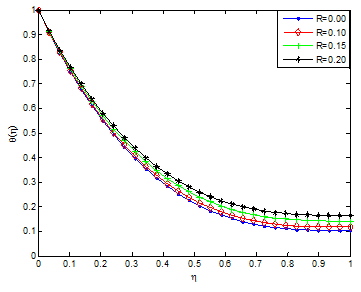Figure 2B Effects of radiation parameter on temperature profile of the Cu-kerosene casson-carreau nanofluid.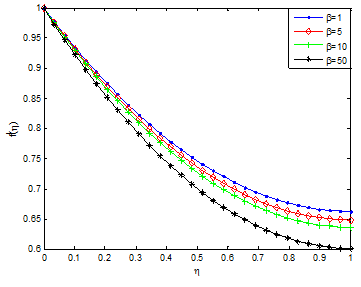Figure 3A Effects of casson parameter on the velocity profile of Ag-kerosene casson-carreau nanofluid.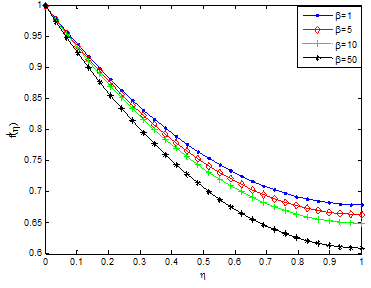Figure 3B Effects of casson parameter on temperature profile of Cu-kerosene casson-carreau nanofluid.Figure 4 Effect of magnetic field parameter (Hartmann number) on the fluid velocity distribution.Figure 5 Effect of magnetic field parameter (Hartmann number) on the fluid temperature distribution.

The effects of unsteadiness parameter on velocity and temperature profiles are shown in Figure 6 & Figure 7, respectively. It is observed that increasing values of S increases the velocity field while decreases the temperature field. This is because as the rate of heat loss by the thin film increases as the value of unsteadiness parameter increases. Figure 8 & Figure 9 depict the effects of Weissenberg number (We) on the velocity and temperature profiles. It is shown from the figures that the velocity increases for increasing values of We and opposite trend was observed in temperature field. The observed trends in the velocity and temperature fields are due to the fact that a higher value of We will reduce the viscosity forces of the Carreau fluid. Increasing the Weissenberg number reduces the magnitude of the fluid velocity for shear thinning fluid while it arises for the shear thickening fluid. The influence of aligned angle on velocity and temperature profiles is presented in Figure 10 & Figure 11. From the figures, it is shown that as the value of aligned parameter increases, the velocity field increases while temperature field decreases.Figure 6 Effect of unsteadiness parameter on the fluid velocity distribution.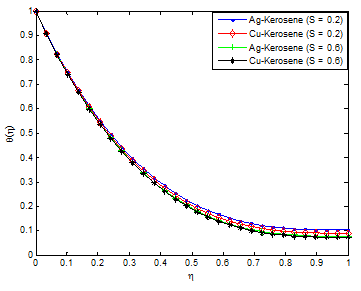Figure 7 Effect of unsteadiness parameter on the fluid temperature distribution.Figure 8 Effect of Weissenberg number on the fluid velocity distribution.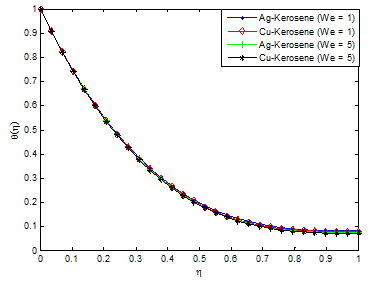Figure 9 Effect of Weissenberg number on the fluid temperature distribution.Figure 10 Effect of aligned angle on the fluid velocity distribution.Figure 11 Effect of aligned angle on the fluid temperature distribution.

Figure 12 & Figure 13 demonstrated the effect of power law index on velocity and temperature fields. As the power index is increased, it was observed that the velocity profile increases while the temperature profile decreases. This is because, increasing value of the power law index, thickens the liquid film associated with an increase of the thermal boundary layer. An increase in the momentum boundary layer thickness and a decrease in thermal boundary layer thickness is observed for the increasing values of the power law index including shear thinning to shear thickening fluids. Also, it should be pointed out that an increase in Weissenberg number correspond a decrease in the local skin friction coefficient and the magnitude of the local Nusselt number s decreases when the Weissenberg number increases. The effects of nanoparticles volume fraction on the velocity and temperature profiles are depicted in Figure 14 & Figure 15, respectively. The result shows that as the solid volume fraction of the film increases both the velocity and temperature field increases. This is because as the nanoparticle volume increases, more collision occurs between nanoparticles and particles with the boundary surface of the plate and consequently the resulting friction enhances the thermal conductivity of the flow and gives rise to increase the temperature within the fluid near the boundary region.Figure 12 Effect of power-law index on the fluid velocity distribution.Figure 13 Effect of power-law index on the fluid temperature distribution.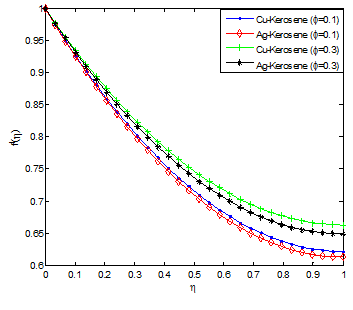Figure 14 Effect of nanoparticle volume fractions on the fluid velocity distribution.Figure 15 Effect of nanoparticle volume fractions on the fluid temperature distribution.

Figure 16 & Figure 17 depict the influence of non-uniform heat source/sink parameter on the temperature field. It is revealed that increasing the non-uniform heat source/sink parameter enhances the temperature fields. It is observed in the analysis that the temperature and thermal boundary layer thickness is depressed by increasing the Prandtl number Pr. The effect of Eckert number on temperature profile is shown in Figure 18. It was established that as the values of Eckert number increases, the values of the temperature distributions in the fluid increases. This is because as Ec increases, heat energy is saved in the liquid due to the frictional heating. The effect of nanoparticle volume fraction ϕ on the film thickness of the nanofluid is shown in Figure 19. It is evident from the figure that the film thickness is enhanced as the values of ϕ is increased. It can be inferred from Eq. (12) that if nanoparticle volume fraction ϕ is increased, the nanofluid viscosity will increased as there exist a direct relationship or proportion between the two parameters. As a result, the increasing viscosity resists the fluid motion along the stretching direction leading to the slowdown of the film thinning process.96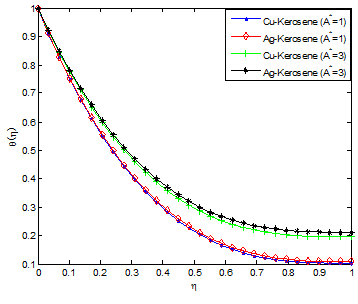Figure 16 Effect of non-uniform heat source/sink parameter (A*) on the fluid temperature distribution.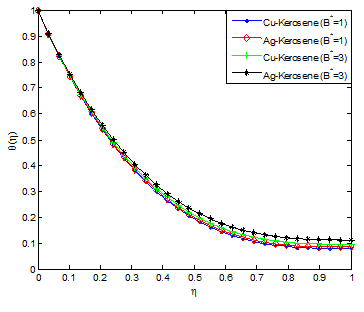Figure 17 Effect of non-uniform heat source/sink parameter (B*) on the fluid temperature distribution.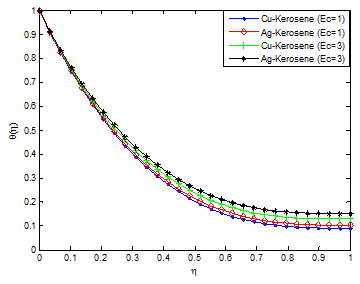Figure 18 Effect of Eckert number on the fluid temperature distribution.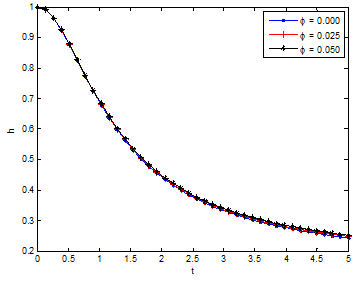Figure 19 Variation of film thickness h with time t for different values of ϕ.

# Conclusion

In this paper, combined influences of thermal radiation, inclined magnetic field and temperature-dependent internal heat generation on unsteady two-dimensional flow and heat transfer analysis of dissipative Casson-Carreau nanofluid over a stretching sheet embedded in a porous medium have been investigated examined. Using kerosene as the base fluid embedded with the silver (Ag) and copper (Cu) nanoparticles, the effects of other pertinent parameters on flow and heat transfer characteristics of the nanofluids are investigated and discussed. From the results, it was established temperature field and the thermal boundary layers of Ag-kerosene nanofluid are highly effective when compared with the Cu-kerosene nanofluid. Thermal and momentum boundary layers of Cu-kerosene and Ag-kerosene nanofluids are not uniform. Heat transfer rate is enhanced by increasing in power-law index and unsteadiness parameter. Skin friction coefficient and local Nusselt number can be reduced by magnetic field parameter and they can be enhanced by increasing in aligned angle. Friction factor is depreciated and the rate of heat transfer increases by increasing the Weissenberg number. This analysis can help in expanding the understanding of the thermo-fluidic behaviour of the Carreau nanofluid over a stretching sheet. Also, the present study has numerous applications involving heat transfer and other applications such as chemical sensors, biological applications, glass, solar energy transformation, electronics, petrochemical products, light-weight, heat-insulating and refractory fiberboard and metallic ceramics etc

None.

# Conflicts of Interest

The authors declare that there is no conflict of interest regarding the publication of this paper.

# Nomenclature

$\stackrel{˙}{a}$ Time-dependent rate

A* Non-uniform heat generation/absorption parameter

B* Non-uniform heat generation/absorption parameter

Bo Electromagnetic induction

Ec Eckert number

M Hartmann number/magnetic field parameter

n Power law index

Pr Prandtl number

$\overline{p}$  Pressure

Re Permeation Reynolds number

$t$  Time

$\overline{u}$ Velocity component in x-direction

$\overline{v}$ Velocity component in y-direction

${U}_{w}$  Fluid inflow velocity at the wall

We Weissenberg number

$\overline{x}$ Coordinate axis parallel to the channel walls

$\overline{y}$  Coordinate axis perpendicular to the channel walls

# Symbol

${\rho }_{nf}$

Density of the nanofluid

${\rho }_{f}$  Density of the fluid

${\mu }_{nf}$ Dynamic viscosity of the nanofluid

${\rho }_{s}$ Density of the nanoparticles

$\varphi$  Fraction of nanoparticles in the nanofluid

$\sigma$ Electrical conductivity
Γ Time constant
γ Aligned angle

# References©2018 Sobamowo, et al. This is an open access article distributed under the terms of the, which permits unrestricted use, distribution, and build upon your work non-commercially.